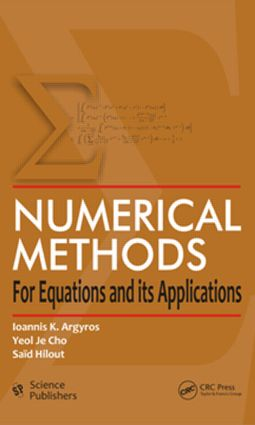Numerical Methods for Equations and its Applications

1st Edition

CRC Press

474 pages

Purchasing Options:\$ = USD
Hardback: 9781578087532
pub: 2012-06-05
SAVE ~\$24.00
\$120.00
\$96.00
x
eBook (VitalSource) : 9780429086427
pub: 2012-06-05
from \$28.98

FREE Standard Shipping!

Description

This book introduces advanced numerical-functional analysis to beginning computer science researchers. The reader is assumed to have had basic courses in numerical analysis, computer programming, computational linear algebra, and an introduction to real, complex, and functional analysis. Although the book is of a theoretical nature, each chapter contains several new theoretical results and important applications in engineering, in dynamic economics systems, in input-output system, in the solution of nonlinear and linear differential equations, and optimization problem.

INTRODUCTION

NEWTON’S METHOD

Convergence under Fréchet differentiability. Convergence under twice Fréchet differentiability. Newton’s method on unbounded domains. Continuous analog of Newton’s method. Interior point techniques. Regular smoothness. ω-convergence. Semilocal convergence and convex majorants. Local convergence and convex majorants. Majorizing sequences.

SECANT METHOD

Convergence. Least squares problems. Nondiscrete induction and Secant method. Nondiscrete induction and a double step Secant method. Directional Secant Methods. Efficient three step Secant methods.

STEFFENSEN’S METHOD

Convergence

GAUSS-NEWTON METHOD

Convergence. Average-Lipschitz conditions.

NEWTON-TYPE METHODS

Convergence with outer inverses. Convergence of a Moser-type Method. Convergence with slantly differentiable operator. A intermediate Newton method.

INEXACT METHODS

Residual control conditions. Average Lipschitz conditions. Two-step methods. Zabrejko-Zincenko-type conditions.

WERNER’S METHOD

Convergence analysis

HALLEY’S METHOD

Local convergence

METHODS FOR VARIATIONAL INEQUALITIES

Subquadratic convergent method. Convergence under slant condition. Newton-Josephy method.

FAST TWO-STEP METHODS

Semilocal convergence

FIXED POINT METHODS

Successive substitutions methods